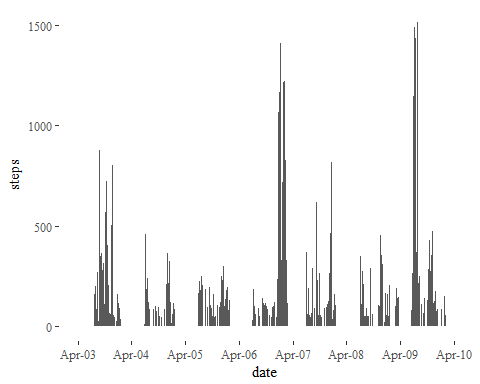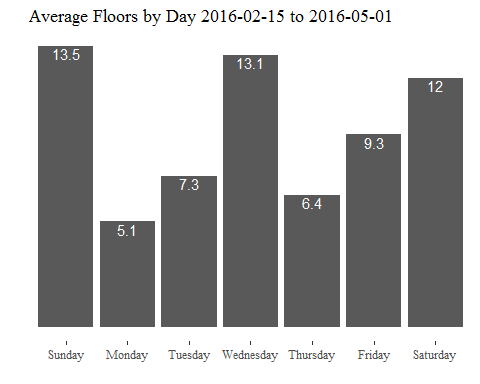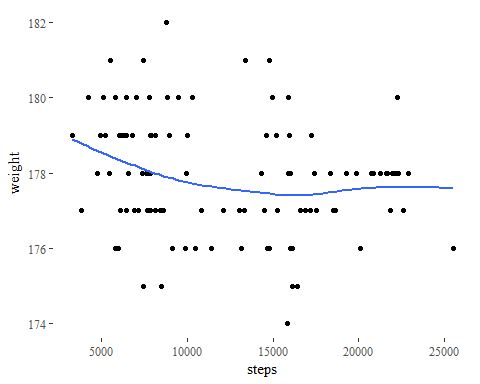# fitbitScraper Examples

## Installation

Install fitbitScraper as you would a normal library. It exists on CRAN, so a simple install.packages("fitbitScraper") should work.

A development version exists on Github, and can be installed via devtools. devtools::install_github("corynissen/fitbitScraper")

## Usage

I’ve stored my password in an environment variable called “FBPW”. I’ll use this to login to fitbit and generate a cookie that will be used for the subsequent requests. You can type it directly in the password field, but it is generally a best practice to use an environment variable instead. Fitbit allows login via Google and Facebook. This library only works with an email / password based login.

library("fitbitScraper")
cookie <- login(email="corynissen@gmail.com", password=Sys.getenv("FBPW"))

Now you can run any of the other functions to get your data. Let’s start with getting steps on a 15 minute interval for a given week…

dates <- seq(as.Date("2016-04-03"), as.Date("2016-04-09"), by="day")
df_list <- lapply(dates, function(x)
df <- do.call(rbind, df_list)

library("ggplot2")
library("ggthemes")
ggplot(df) +
geom_bar(aes(x=time, y=steps), stat="identity") +
theme_tufte() +
scale_x_datetime(name="date", date_breaks="1 day", date_labels="%b-%d")You can get a daily summary of your data also. Here, I download data for the number of flights of stairs I climbed for the last two months. Then, compute the average number of flights of stairs by day, and graph it. Not surprisingly, I climb more stairs on the weekends when I’m home than I do at work during the week. Note that it is possible to get this data using get_intraday_data() for each day, but this is much more efficient using just one call to the fibit API for the entire date range instead of one API call per day.

df <- get_daily_data(cookie=cookie, what="floors", start_date="2016-02-15",
end_date="2016-05-01")
df$weekday <- format(df$time, "%A")
avgs <- by(df$floors, df$weekday, mean)
avgs <- data.frame(day=names(avgs), floors=as.numeric(avgs))
avgs$day <- factor(avgs$day, levels=avgs$day[c(4, 2, 6, 7, 5, 1, 3)]) ggplot(avgs) + geom_bar(aes(x=day, y=floors), stat="identity") + theme_tufte() + xlab("") + ylab("") + ggtitle("Average Floors by Day 2016-02-15 to 2016-05-01") + geom_text(aes(x=day,y=floors,label=round(floors, 1)), vjust=1.1, colour="white") + theme(axis.text.y=element_blank(), axis.ticks.y=element_blank()) + theme(plot.title=element_text(vjust=.5))Another thing to look at, especially if you have the Aria scale, is your weight. You can record your weight manually in the fitbit app, which is how I do it, or the Aria scale will sync it automatically. Any how, let’s graph my steps vs. weight for a time period and see if there seems to be a correlation. Data is returned for use in a graph on the fitbit page, so if you include a date range larger than two weeks or so, it returns data for a subset of the days in your range. Fitbit seems to play pretty loose with the start and end dates too. I want data from 2015-01-01 to 2015-05-01, so I’ll break that into several requests to fitbit. # don't do this... # mywt <- get_weight_data(cookie, start_date="2015-01-01", end_date="2015-05-01") start_date <- as.Date("2015-01-01") end_date <- as.Date("2015-05-01") wt_df_list <- list() # initialize a list to put the weight dataframes into in_range <- TRUE # indicator variable to tell when to exit while loop s_date <- start_date # date to start with during loop while(in_range){ e_date <- s_date + 14 new_df <- get_weight_data(cookie, start_date=as.character(s_date), end_date=as.character(e_date)) wt_df_list[[as.character(s_date)]] <- new_df s_date <- e_date + 1 if(e_date > end_date) in_range <- FALSE } wt_df <- do.call(rbind, wt_df_list) wt_df <- wt_df[!duplicated(wt_df$time), ]
wt_df <- wt_df[order(wt_df$time), ] wt_df <- wt_df[as.Date(wt_df$time) >= start_date &
as.Date(wt_df$time) <= end_date, ] step_df <- get_daily_data(cookie=cookie, what="steps", start_date="2015-01-01", end_date="2015-05-01") # get common date format to merge data sets... wt_df$date <- as.character(as.Date(wt_df$time)) step_df$date <- as.character(as.Date(step_df\$time))

# merge by date
df <- merge(wt_df, step_df, by="date")

# now plot
ggplot(df, aes(x=steps, y=weight)) +
geom_point() +
stat_smooth(se=FALSE) +
theme_tufte()That last example illustrates one of the limitations of retrieving the data the way this library does. Instead of using the “official” fitbit API, this library uses the API intended for their website developer to use to build the visualizations on the web dashboard. So, there’s no public documentation. This results in situations like the last one where the weight data returned by that API call is intended to be used in a chart, so they don’t need more than 20 points, so that’s all that is returned, no matter how large the date range supplied is. Keep this in mind when you request data and use caution that you are being returned data for the same dates that you requested it for.